In the verge of coronavirus pandemic, we are providing FREE access to our entire Online Curriculum to ensure Learning Doesn't STOP!

# Measuring Weight

Go back to  'Mensuration and Measurement'

## Measurement of weight

The concept of measurement begins by making children understand the different parameters on which objects are measured. In Grade K children learn how an object can be described using its length, weight and capacity using simple terms like heavy & light, tall & short, big & small. An understanding of these concepts would set the stage for understanding the measurement of objects using standardised units.

The image shows a comparison of the weights. A lion is heavier than the monkey.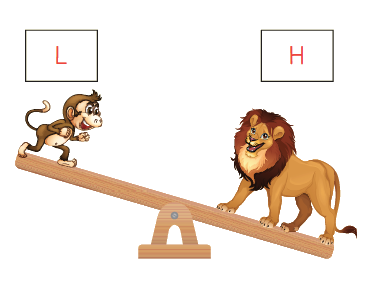## Non-standard units of measuring weight

Non-standard units of measurement are used in early years of learning, to introduce children to the concept of measuring without them having to read any scales. Reading scales of any kind is a difficult skill in itself, so the idea of non-standard measures is to focus the child on the concept of heavier, lighter, longer, shorter, etc. before they move onto the next step of measuring using standard units.

To measure weight, You can give the child two fruits a strawberry and a banana. Then you can ask them questions like what feels lighter? or what feels heavier? This can also be explained using a weighing balance.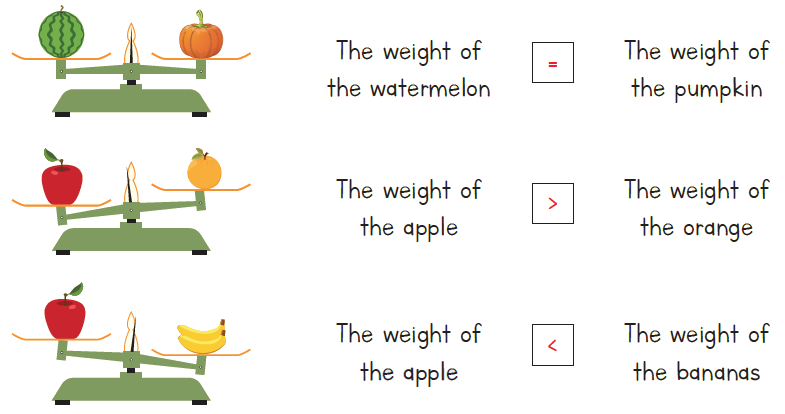Give them some blocks and ask them to keep it on the weighing scale to see what is and their compare the weight. This would also help them see how they can balance the weighing scale by keeping the same number of blocks on both sides. It will help build an intuitive understanding of measuring weight.

## Standard units to measure weight

Children learn to use standard units of measurement in Grade 2, They learn about the tools and units appropriate for different objects. For example: The weight of an object can be measured using different types of weighing scales. These weighing scales use standard units such as milligrams, grams and kilograms to measure the weight of different objects. The weighing scales can be battery run or manual. The weighing that are operated on manually use weights as shown below.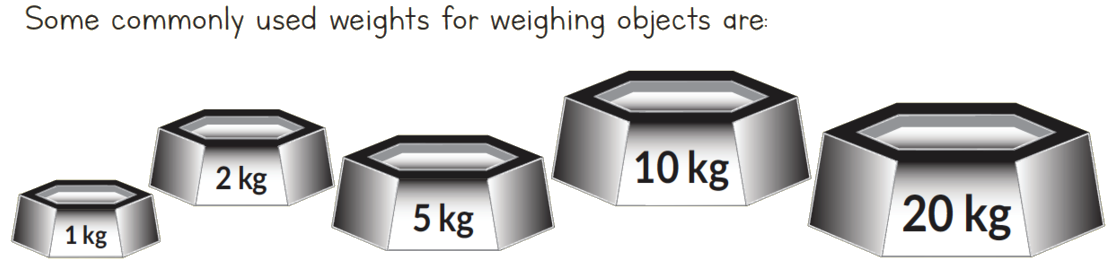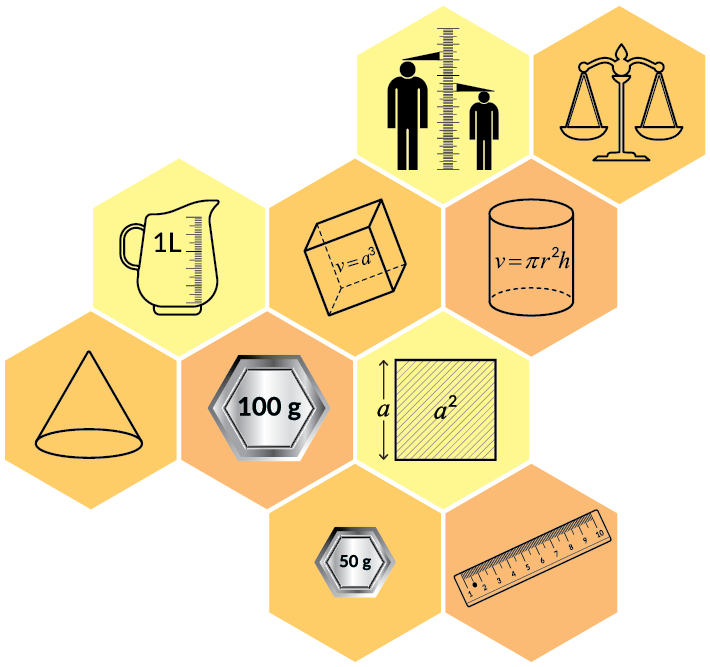### Formula

Children also learn about the relationship between units of measurement. They would need to know the following facts:

1000 milligrams = 1 gram (gm)
1000 grams = 1 kilogram(kg)

Using this fact, they would be able to convert the weights. Add and subtract weights. A thorough understanding of the conversion of units can be built by exposing children to real life situations where measuring converting and figuring out the solution happens.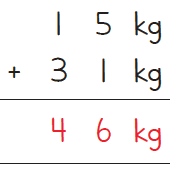Children would continue this kind of problem-solving in until Grade 5. They would solve application based problems involving all four operations (addition, subtraction, multiplication and division).

## Tips and tricks

• Tip: Children often forget if they have to multiply or divide by 1000 to convert from kg to g. But children find it easy to remember and visualise that 1 kilogram is a bigger and heavier block than a block of 1 gram. Use this visualisation to help with conversion. If the same kilogram needs to be constructed using grams, you’ll need many small blocks. Help children recall that to get a larger number we multiply. So when converting from kg to g, we multiply by 1000.
• Compared to the base unit (meters for length, grams for weight), “kilo” is always a factor of 1000 larger. And “milli” is always a factor of 1000 smaller. Seeing the parallel between weight and length measures helps. This way you have to remember just one because the other will have a similar conversion factor.
• A helpful trick is to remember your own weight in grams and in kilograms. Needless to say your weight in grams will be a large number. This large numerical value helps children remember that when converting from kg to g, the numerical value will be quite large.
Measurement of weight and capacity
Measurement of weight and capacity
A Visual Introduction to Measurement
Kg | Worksheet 1
A Visual Introduction to Measurement
Kg | Worksheet 2
An Introduction to Measurement